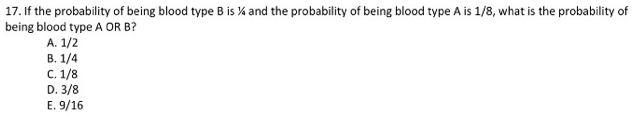# Problem: If the probability of being blood type B is 1/4 and the probability of being blood type A is 1/8, what is the probability of being blood type A or B?A. 1/2B. 1/4C. 1/8D. 3/8E. 9/16

🤓 Based on our data, we think this question is relevant for Professor Montgomery's class at NAU.

###### FREE Expert Solution

In blood types, the probability of having blood type A is independent of the probability of having blood type B. Furthermore, the blood type O cannot be seen as long as either of these two are included as an allele.###### Problem Details

If the probability of being blood type B is 1/4 and the probability of being blood type A is 1/8, what is the probability of being blood type A or B?

A. 1/2

B. 1/4

C. 1/8

D. 3/8

E. 9/16